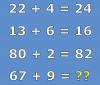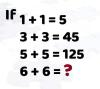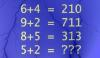BRAIN TEASERSBrain Teasers User Profile

# Ognjen Cvetkovic

rank
252
points
16
See full ranking list
short ranking list
 250 Chatchai Khumtaveeporn 16 251 VAHID KHALILI 16 252 Ognjen Cvetkovic 16 253 Boreli Dejan 15 254 nichelle ainsley 15
 Mathematical Puzzle: IF 22+4... Mathematical Puzzle: IF 22+4=24, 13+6=16 and 80+2=82 THEN 67+9=?Mathematical Puzzle: IF 1+1=... Mathematical Puzzle: IF 1+1=5, 3+3=45 and 5+5=125 THEN 6+6=?Mathematical Puzzle: IF 6+4=... Mathematical Puzzle: IF 6+4=210, 9+2=711 and 8+5=313 THEN 5+2=?